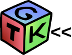gtkIOStream  1.7.0 GTK+ << C++ IOStream operators for GTK+. Now with ORBing, numerical computation, audio client and more ...
NeuralNetwork< TYPE > Class Template Reference

`#include <NeuralNetwork.H>`

## Public Member Functions

NeuralNetwork (void)
Constructor. More...

virtual ~NeuralNetwork (void)
Destructor. More...

void activate (vector< NeuralLayer< TYPE > *> &layers, Eigen::Matrix< TYPE, Eigen::Dynamic, 1 > &input)

## Detailed Description

### template<typename TYPE> class NeuralNetwork< TYPE >

Implements a feed forward neural network. The network is used as follows :

// Begin constructing the neural network topology
vector<NeuralLayer<double> *> networkLayers;
networkLayers.push_back(new TanhLayer<double>(weights, bias));
networkLayers.push_back(new TanhLayer<double>(weights, bias));
// setup some input
Eigen::Matrix<double, Eigen::Dynamic, 1> input(10,1);
input<<0.8333,0.8333,0.8333,0.8333,0.8333,0.6871,0.5833,0.4371,0.3333,0.4000;
// Actiave the Neural Network
nn.activate(networkLayers, input);
// the result is in the last layer
cout<<networkLayers[networkLayers.size()-1]->output<<endl;
Template Parameters
 TYPE the precision of the data to use, e.g. float, double
Examples:
NeuralNetworkFnTest.C, and NeuralNetworkTest.C.

Definition at line 222 of file NeuralNetwork.H.

## ◆ NeuralNetwork()

template<typename TYPE>
 NeuralNetwork< TYPE >::NeuralNetwork ( void )
inline

Constructor.

Definition at line 225 of file NeuralNetwork.H.

## ◆ ~NeuralNetwork()

template<typename TYPE>
 virtual NeuralNetwork< TYPE >::~NeuralNetwork ( void )
inlinevirtual

Destructor.

Definition at line 228 of file NeuralNetwork.H.

## ◆ activate()

template<typename TYPE>
 void NeuralNetwork< TYPE >::activate ( vector< NeuralLayer< TYPE > *> & layers, Eigen::Matrix< TYPE, Eigen::Dynamic, 1 > & input )
inline

Activates all layers in the neural network. The last layer has the output

Parameters
 layers Various neural network layers, 0 being the input layer input The input vector to feed forward

Definition at line 235 of file NeuralNetwork.H.

The documentation for this class was generated from the following file:
gtkIOStream: NeuralNetwork< TYPE > Class Template Reference
 Support this project by purchasing our hardware. GTK+ IOStream  Beta